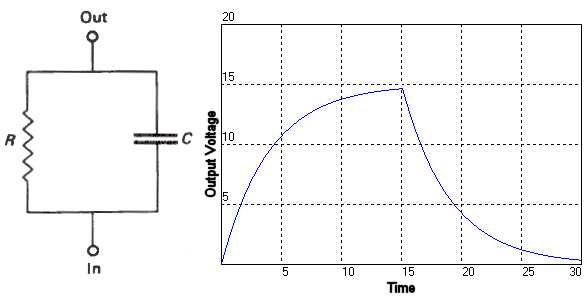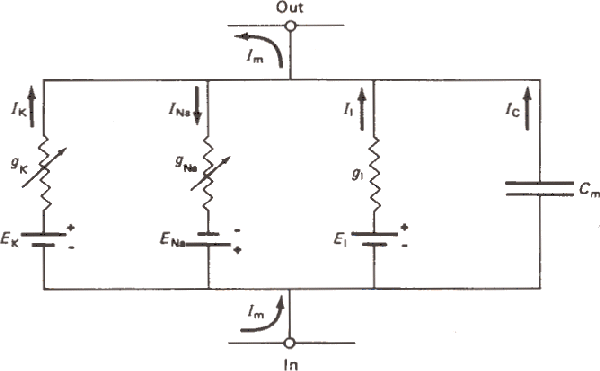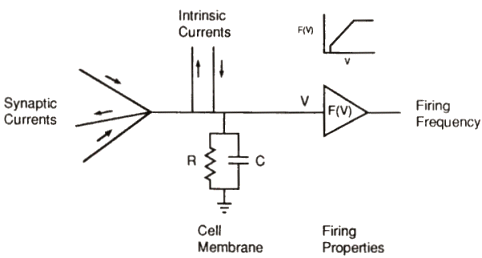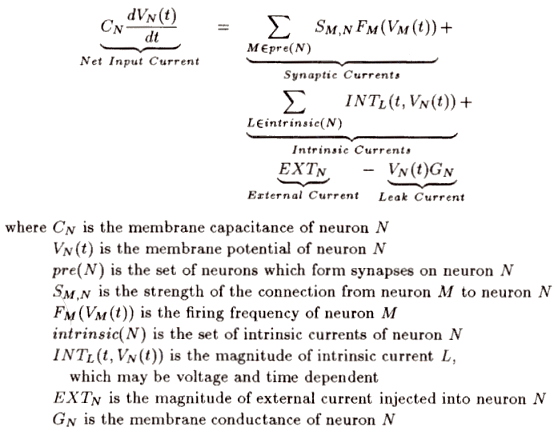# Neuron Model### Electrical Equivalent Circuit

In the Neuron Basics section it was shown that the membrane potential is controlled by the inflow and outflow of ions. In fact, the triggering of the action potential is caused when neurotransmitters open passive transports allowing these ions to flow in or out of the neuron. This in turn changes the potential across the cell. This type of interaction can be modeled in an electrical equivalent circuit using a battery and a resistor. The battery represents the stored potential that is maintained by the sodium/potassium pumps, and the resistor (or conductor) represents the quantity of ions that are allowed to flow in or out of the cell. The more transports that are opened, the more ions flow. Or in other words, the resistance to flow for the ions is decreased, conductance is increased, by having more transports open. So the passive transports are basically just variable resistors. Figure 2 shows the equivalent electrical circuit for a neuron. In that circuit it can be seen that the K+ and Na+ transports are shown as variable resistors, and that the batteries associated with each are reversed. The reason for this is that the sodium is most concentrated outside the cell, and potassium is most concentrated inside the cell. This means that current that is generated from the potassium transports flow out of the cell and currents from sodium transports flow into the cell. However, the flow of current from the passive transports are not the only piece to the puzzle. Another important contributor to the electrical behavior of the cell is the charge separation that is maintained across the cell membrane. This separation of charge by an insulating material causes a capacitive affect on the cell. Figure 1 shows a simple RC circuit. If a voltage is applied to this circuit then the capacitor begins storing the electricity. Eventually it will reach its maximum storage capacity and simply stay there. Once the external voltage is removed however the capacitor then begins to discharge its stored power across the resistor until it has been fully discharged. The important thing to see here is that the voltage across the capacitor resists change. For example, if this circuit had only a resistor in it then as soon as the power was applied the voltage would change to its steady state value, and when removed it would then go to zero immediately. But the capacitor resists the change because it takes time for it to charge and discharge. Neurons also display this type of electrical behavior. Figure 2 shows the neural equivalent circuit with a capacitor that is in parallel with the other variable resistors to model this property.Figure 1. RC Circuit and output voltage characteristics.Figure 2. This is the neuron equivalent circuit. INa and Ik are the currents for the sodium and potassium transports. Ii is the intrinsic current. Ic is the current from the membrane capacitance. (Beer, 1990)

### Intrinsic Currents

The equivalent circuit in figure 2 also has a current which has not yet been explained called the Ii current. This is the intrinsic current of the neuron. Neurons are very dynamic objects. Some types of  neurons called pacemakers are capable of spontaneously firing in a rhythmic fashion, others can fire randomly. These behaviors are caused by very complex and highly dynamic properties within the neurons. However, the behavior they produce are often relatively simple to model. Intrinsic currents are simply a way to model this complex behavior with a simple mechanism. For example, in order to model a randomly firing neuron all that needs to be done is to use a model for a regular neuron and randomly inject that neuron with current. Intrinsic is meant to imply that these random currents are generated by the neuron themselves and are not really being injected from outside the cell. Also, the sensory neurons use the intrinsic currents extensively. For instance, if you want to model a neuron that senses the rotation of a leg you would map the radian rotation of the leg to intrinsic currents using an equation. Then, any rotational angle would be associated with a given intrinsic current and that would affect the rate of fire of the neuron. Basically all the intrinsic currents are is a modeling trick to make neurons with more complex behaviors.

### Model DescriptionFigure 3. This is a diagram showing the basic elements of the model of the neuron. (Beer, 1990)

Figure 3 shows all of the basic elements that are used to simulate a model neuron. On the far left, synaptic currents are shown going into and out of the cell. These are the currents coming from other neurons that are firing. Next are the intrinsic currents. These are used to emulate complex behaviors of the neuron and are used for sensory neurons. The basic RC circuit comes next. Finally, the model neuron uses an equation that relates the current membrane potential to the overall firing rate of the neuron. The model used in this system does not explicitly simulate every single action potential, it only simulates the overall rate of fire for the neuron at a given moment in time. This is done in an attempt to simplify the simulation while still maintaining the important dynamic character of the neuron. The equation used for this comes from the experiments discussed earlier that directly related the input current to the firing rate. However, voltage is being used here instead of input current. This is justified because the rapid voltage swings associated with action potentials are being ignored. There are three parameters associated with the equation. The first is the minimum firing threshold. Below this voltage level the neuron does not fire at all. The next is the minimum firing frequency. And the third is the gain of the firing function. This is basically the slope of the line in the equation. The firing rate can not go above one or below zero.

### Model EquationsFigure 4. Neural model equation. (Beer, 1990)

Figure 4 shows the mathematical equation that describes the equivalent circuit from Figure 2. The mathematical relationship between the voltage and current of a RC circuit is a differential equation. The synaptic current term is the summation of all of the input currents entering this neuron from other synapses. SM,N is the synaptic strength of the axon from neuron M to this neuron N. FM is the firing rate equation that relates the current membrane voltage to the rate of fire of neuron M. So the input current from neuron M is the synaptic strength of the axon connecting neurons M and N, times the current firing frequency of neuron M. The intrinsic current term is the summation of all of the intrinsic currents for this neuron. The external current is any current that being injected from outside the cell. For example, an experimenter might want to temporarily disable a neuron by injecting it with a strong inhibiting current. The leak current is a term that accounts for the current that is leaked from the neuron out of the cell via the resistor in the RC circuit. The equation shown above is solved using Euler's method of numerical integration. (for details click here.) This gives a value for the membrane voltage of a neuron at a specific point in time.

### Synapse Model

The other major portion of the neuron model used in the simulation that needs to be discussed is the various synapses that are used. The regular synapse simply multiplies the synapse strength times the firing frequency of the origin neuron to get the synaptic current. However, there are two other synapse types that are used. Both of these model synaptic behavior that is seen in real systems. The first is the gated synapse. This type of synapse acts as a gate to either turn on or off the current from another synapse into a given neuron. The equation for this is I = (U + Sign(IG))IS .Where U is the un-gated state of the synapse, IG is the synaptic current of the gating synapse, and IS is the current of the gated synapse. So for instance if U is one and IG is negative then the current IS never goes into the neuron, but if IG is zero then IS does go into the neuron. The next type of synapse is modulated. Gated synapses are like an all or nothing switch, but modulated synapses are like a light dimmer or an amplifier. The equation for modulated synapses is:

(1 + IM)IS  if IM > 0
IS/(1+ |IM|) if IM < 0

So if the modulating current is greater than zero then it amplifies the synaptic current. And if the modulating current is less than zero then it reduces the synaptic current. These two types of synapses allow one neuron to control synaptic connections between two other neurons. This is a very important feature that is used several times in the insect simulation system.